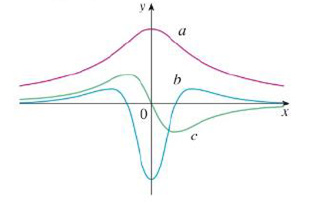Chapter 2, Problem 48RE### Single Variable Calculus: Early Tr...

8th Edition
James Stewart
ISBN: 9781305270343

#### Solutions

Chapter
Section### Single Variable Calculus: Early Tr...

8th Edition
James Stewart
ISBN: 9781305270343
Textbook Problem

# The figure shows the graphs of f, f', and f". Identify each curve, and explain your choices.To determine

To Identify: The curves f,f and f on given with proper explanation.

Explanation

Graph:

The given graph is shown as in Figure 1,

Observation:

From the Figure 1, it is observed that the point b(x)=0 is the same point where the graph of c has horizontal tangents.

Recall that the derivative of a function is zero where the function has a horizontal tangent.

Therefore, b(x) is the derivative of the graph c(x). That is, c(x)=b(x).

Observe the graph of a and c carefully.

The slope of the curve is positive for x<0 and the slope is negative for x>0. c is the only curve which is positive or x<0 and negative for x>0

### Still sussing out bartleby?

Check out a sample textbook solution.

See a sample solution

#### The Solution to Your Study Problems

Bartleby provides explanations to thousands of textbook problems written by our experts, many with advanced degrees!

Get Started

#### Find more solutions based on key concepts